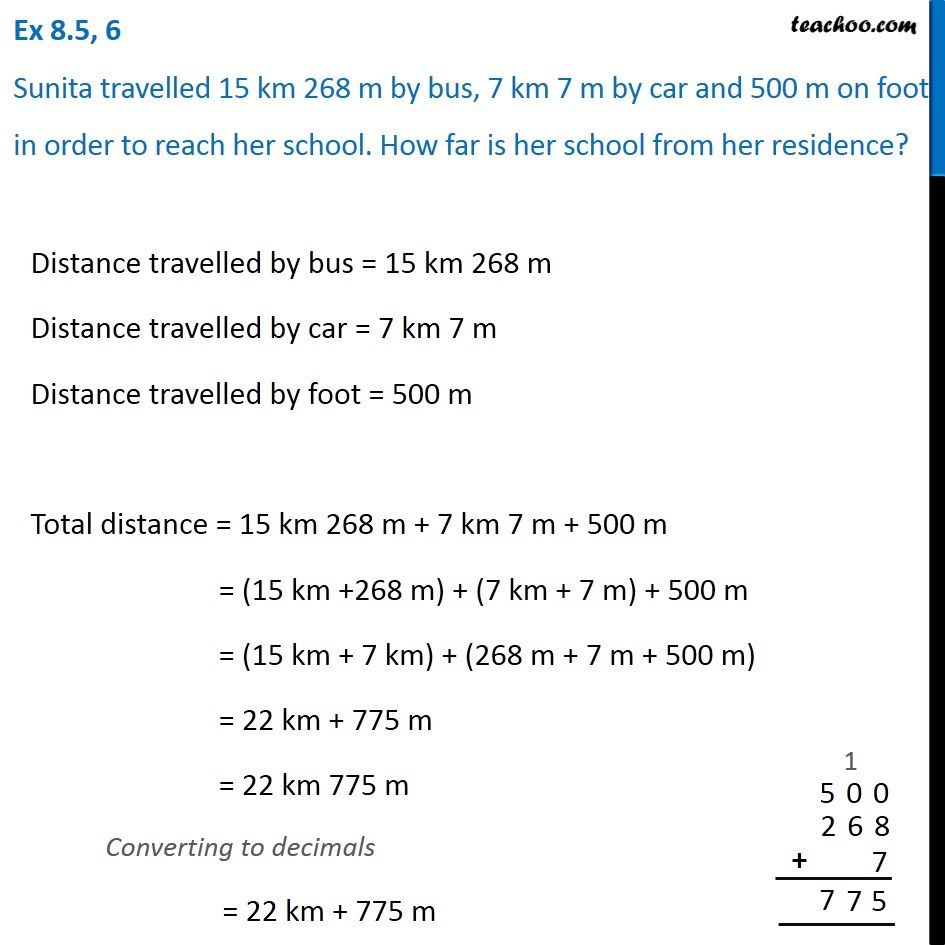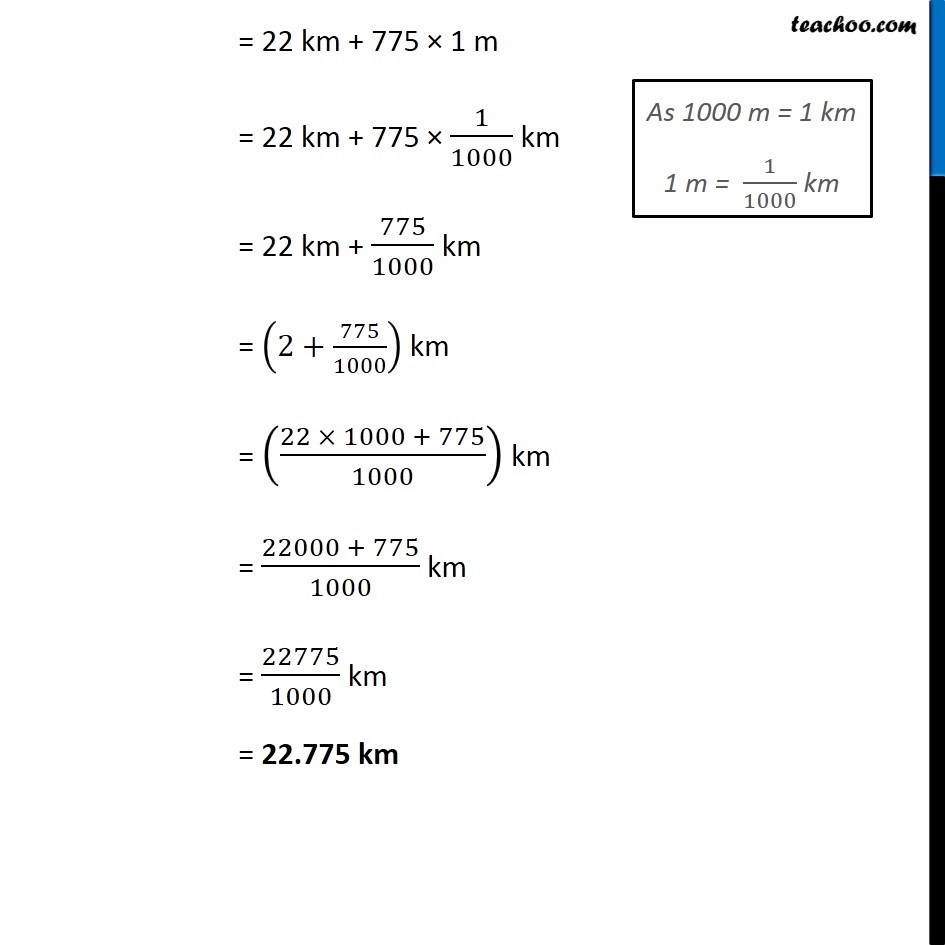Addition of decimal numbers - Statement questions

Chapter 8 Class 6 Decimals
Concept wise### Transcript

Ex 8.5, 6 Sunita travelled 15 km 268 m by bus, 7 km 7 m by car and 500 m on foot in order to reach her school. How far is her school from her residence?Distance travelled by bus = 15 km 268 m Distance travelled by car = 7 km 7 m Distance travelled by foot = 500 m Total distance = 15 km 268 m + 7 km 7 m + 500 m = (15 km +268 m) + (7 km + 7 m) + 500 m = (15 km + 7 km) + (268 m + 7 m + 500 m) = 22 km + 775 m = 22 km 775 m Converting to decimals = 22 km + 775 m = 22 km + 775 × 1 m = 22 km + 775 × 1/1000 km = 22 km + 775/1000 km = (2+775/1000) km = ((22 × 1000 + 775)/1000) km = (22000 + 775)/1000 km = 22775/1000 km = 22.775 km